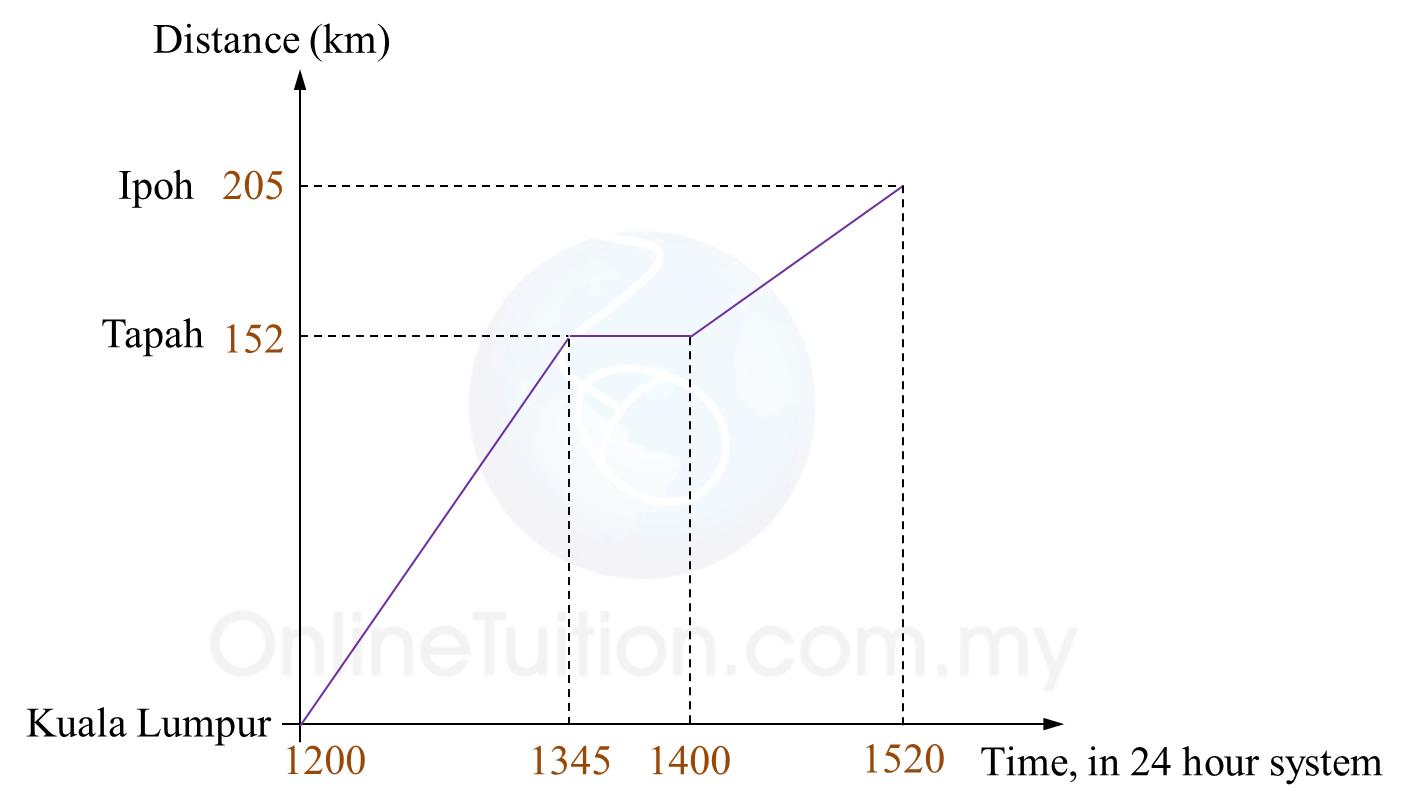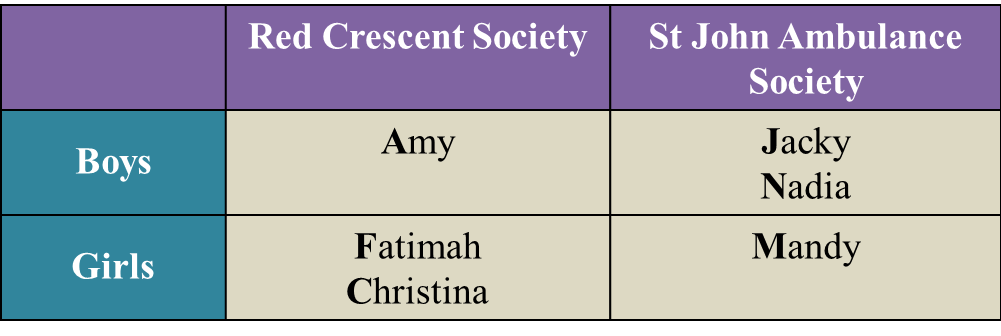# SPM Mathematics 2018, Paper 2 (Questions 9 & 10)

Question 9 (5 marks):
Diagram 7 shows a distance-time graph for the journey of a car from Kuala Lumpur to Ipoh.(a) State the duration of time, in minutes, the driver stopped and rest at Tapah.
(b) Calculate the speed, in kmh-1, of the car from Kuala Lumpur to Tapah.
(c) Calculate the average speed, in kmh-1, of the car for whole journey.

Solution:
(a)
1400 – 1345 = 15 minutes
The driver stopped and rest at Tapah for 15 minutes.

(b)

(c)

Question 10 (5 marks):
The table below shows some members of Red Crescent Society and St John Ambulance Society that are instructed to do an outdoor tasks at various places during the Flag Day.Two members from the societies were dropped off random at those places.
(a) List all the possible outcomes of the event in this sample space.
You also may use the letters such as A for Amy and so on.
(b) By listing down all the possible outcomes of the event, find the probability that
(i) a boy and a girl were dropped off at a certain place.
(ii) both members that were dropped off at a certain place are from the same society.

Solution:
(a)
S = {(A, J), (A, N), (A, F), (A, C), (A, M), (J, A), (J, N), (J, F), (J, C), (J, M), (N, A), (N, J), (N, F), (N, C), (N, M), (F, A), (F, J), (F, N), (F, C), (F, M), (C, A), (C, J), (C, N), (C, F), (C, M),(M, A), (M, J), (M, N), (M, F), (M, C)}

(b)(i)
{(A, F), (A, C), (A, M), (J, F), (J, C), (J, M), (N, F), (N, C), (N, M), (F, A), (F, J), (F, N), (C, A), (C, J), (C, N), (M, A), (M, J), (M, N)}
$\begin{array}{l}\text{Probability}\\ =\frac{18}{30}\\ =\frac{3}{5}\end{array}$

(b)(ii)
{(A, F), (A, C), (F, A), (F, C), (C, A), (C, F), (J, N), (J, M), (N, J), (N, M), (M, J), (M, N)}
$\begin{array}{l}\text{Probability}\\ =\frac{12}{30}\\ =\frac{2}{5}\end{array}$